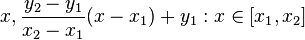# Point (geometry)

A spatial point is a concept used to define an exact location in space. It has no volume, area or length, making it a zero dimensional object. Points are used in the basic language of geometry, physics, vector graphics (both 2D and 3D), and many other fields. In mathematics generally, particularly in topology, any form of space is considered as made up of an infinite amount of points as basic elements.

## Points in Euclidean geometry

In Euclidean geometry, points are one of the fundamental objects. Originally defined by Euclid as "that which has no part," this essentially means that it has no length, width, depth or any higher dimensional measure of value. In two dimensional space, a point is represented by an ordered pair (a1,a2) of numbers, where a1 conventionally represents it's location on the x-axis, and a2 represents it's location on the y-axis. For higher dimensions, a point is represented by a ordered collection of n elements, (a1, a2, ..., an) where n is the dimension of the space.

Euclid both postulated and asserted many key ideas about points. His first postulate is that it was possible to draw a straight line from any point to any other point. This is confirmed in modern day set theory in two dimensions by the set F =${x, \frac{y_2 - y_1}{x_2 - x_1}(x - x_1) + y_1 : x \in [x_1, x_2]}$, with higher dimensional analogues existing for any given dimension. Euclid sometimes implicitly assumed facts that did not follow from the axioms (for example about the ordering of points on lines, and occasionally about the existence of points distinct from a finite list of points). Therefore the traditional axiomatization of point was not entirely complete and definitive.

Observe that there are also approaches to geometry in which the points are not primitive notions. The notion of "region" is primitive and the points are defined by suitable "abstraction processes" from the regions (see Whitehead's point-free geometry].

## Points in topology

In topology, a point is simply an element of the underlying set of a topological space. Similar usage holds for similar structures such as uniform spaces, metric spaces, and so on.

## Inwards Infinitude

The point, being often characterized as "infinitely small," is the geometrical representation of the inwards infinitude, greater natural principle spread throughout every mathematical field, where any finite value, part of a greater infinite value, is itself formed by infinite finite values. Likewise, the point, though immeasurable, is the basic element of any measurable form. It is so for, even having it no dimensions, neither height, width nor length, its association causes the existence of such. (Two zero-dimensional points can form a one-dimensional line; two lines can form a two-dimensional surface; two surfaces can form a three-dimensional object)

As it is, the point, in geometry, is the basic visual (imaginable) representation for the minimal structure of existence.

Measurability of immeasurable elements associations, or limited infinitude, is what makes it, for many people, in common language, so "abstract" and hard to understand (like trying to picture a point), but inwards infinitude appears, for instance, within every irrational number, such as pi, and complies with every rule of existence, matter or not, being the point one possible interpretation of what would be the basis of it.